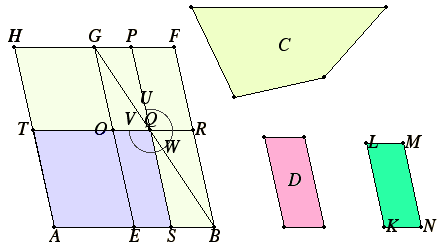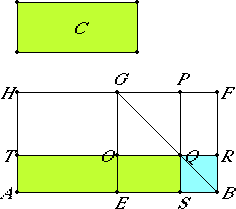# Proposition 28

To apply a parallelogram equal to a given rectilinear figure to a given straight line but falling short by a parallelogram similar to a given one; thus the given rectilinear figure must not be greater than the parallelogram described on the half of the straight line and similar to the given parallelogram.
VI.27

Let C be the given rectilinear figure, AB the given straight line, and D the given parallelogram, and let C not be greater than the parallelogram described on the half of AB similar to the given parallelogram D.

It is required to apply a parallelogram equal to the given rectilinear figure C to the given straight line AB but falling short by a parallelogram similar to D.Bisect AB at the point E. Describe EBFG similar and similarly situated to D on EB, and complete the parallelogram AG.

If then AG equals C, that which was proposed is done, for the parallelogram AG equal to the given rectilinear figure C has been applied to the given straight line AB but falling short by a parallelogram GB similar to D.

But, if not, let HE be greater than C.

Now HE equals GB, therefore GB is also greater than C.

VI.25

Construct KLMN equal to GB minus C and similar and similarly situated to D.

VI.21

But D is similar to GB, therefore KM is also similar to GB.

Let, then, KL correspond to GE, and LM to GF.

Now, since GB equals C and KM, therefore GB is greater than KM, therefore also GE is greater than KL, and GF than LM.

Make GO equal to KL, and GP equal to LM, and let the parallelogram OGPQ be completed, therefore it is equal and similar to KM.

Therefore GQ is also similar to GB, therefore GQ is about the same diameter with GB.

Let GQB be their diameter, and describe the figure.

Then, since BG equals C and KM, and in them GQ equals KM, therefore the remainder, the gnomon UWV, equals the remainder C.

And, since PR equals OS, add QB to each, therefore the whole PB equals the whole OB.

I.36

But OB equals TE, since the side AE also equals the side EB, therefore TE also equals PB.

Add OS to each. Therefore the whole TS equals the whole, the gnomon VWU.

But the gnomon VWU was proved equal to C, therefore TS also equals C.

Therefore there the parallelogram ST equal to the given rectilinear figure C has been applied to the given straight line AB but falling short by a parallelogram QB similar to D.

Q.E.F.

## Guide

When this proposition is used, the given parallelgram D usually is a square. Then the problem is to cut the line AB at a point S so that the rectangle AS by SB equals the given rectilinear figure C. This special case can be proved with the help of the propositions in Book II. See the Guide to proposition II.5 for more details.

#### The outline of a simplified proof for rectangles

The proof of the current proposition is difficult to follow. It is simplified when we take the special case mentioned above, namely, when the given parallelogram D is a square. The simplified proof is easier to follow since the rest of the parallelograms mentioned all become rectangles.The construction is as follows. Bisect AB at E, construct a square GFBE. The next stage is to construct a square GPQO equal to the square GFBE minus the figure C. For an alternate construction of GPQO, see the lemma for X.14 which applies I.47 to do that.) Complete the figure. We can understand the meaning of this construction more easily if we interpret it algebraically. Let a stand for the known quantity AB, and c the known quantity C. Then let x and y stand for the unknown quantities SB and SA. Then this construction finds x and y so that their sum is a and their product is c.

In terms of the single variable x, the construction solves the quadratic equation ax – x2 = C.

The next proposition solves a similar quadratic equation: ax + x2 = C.

#### Use of this proposition

This construction in this proposition is used in propositions X.33 and X.34.